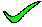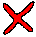There are no repeating terms, so it is not un-normalised. To be more than first normal form, is must not have any partial key dependencies. B->C is a partial key dependency, so it is in first normal form.

closeThere are no repeating terms, so it is not un-normalised. There is a partial key dependency (B->C), so it is not in second normal form.

closeThere are no repeating terms, so it is not un-normalised. There is a partial key dependency (B->C), so it is not in third normal form.

closeThere are no repeating terms, so it is not un-normalised. There is a partial key dependency (B->C), so it is not in BCNF normal form.

closeThere are no repeating terms, so it is not un-normalised. However, there is a partial key dependency (B->C), so it is in first normal form.

close

 A B C D E TELL ME NEXT INDEX

Consider the relational schema R(A,B,C,D,E) with non-key functional dependencies C,D -> E and B -> C.

Select the strongest statement that can be made about the schema R

1. R is in first normal form
2. R is in second normal form
3. R is in third normal form
4. R is in BCNF normal form
5. None of the above